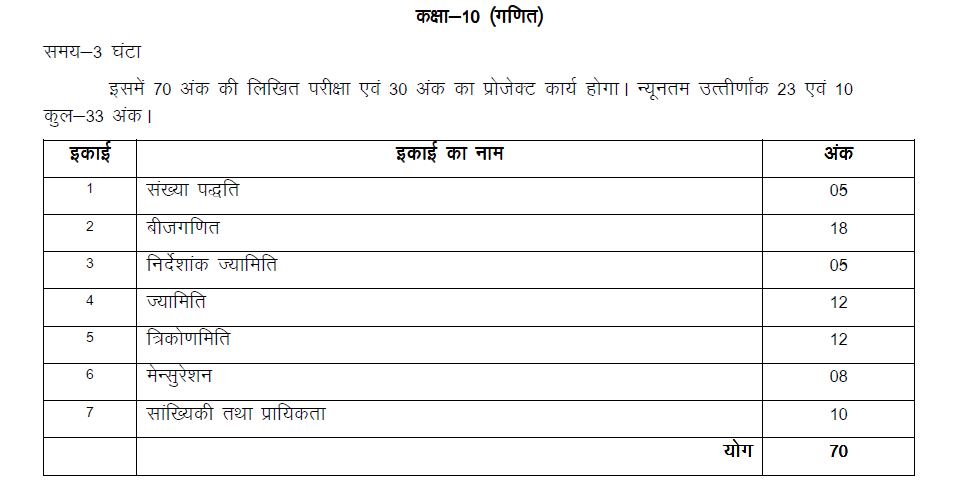UP Class 10 Maths Syllabus

Understanding of math syllabus is very important because you get clarity about the different chapters covered and can plan your study accordingly. Accurate planning will be possible by having a detailed maths up board 1oth syllabus copy handy. Maths syllabus makes you aware of the difficult and easy chapter introduced in arithmetic, algebra, geometry, trigonometry for that particular academic year. Based on the level of difficulty you can dedicate study hours to those chapters. Also, it makes you realize the topics that you should concentrate more. Here we have given the Maths Syllabus For Class 10 UP Board. Students can access the up board class 10 maths solution at BYJU’S to prepare for the exams.

UP Board Class 10 Maths Exam Pattern with Marks Distribution

The topics introduced in the class 10 math are necessary to understand the intermediate math chapters. Students can also solve the up board class 10 model paper to get an idea about question pattern . Know the chapterwise marks distribution of the UP Board Class 10 Ganith Syllabus below:

गणित पाठ्यक्रम का संक्षिप्त विवरण जानेंMaths Syllabus For Class 10 UP Board

The chapters covered in class 10 maths is given in the below table. Students willing to score excellent marks in class 10 UP Board maths exam should be thorough with the maths syllabus.

To download the detailed syllabus of mathematics for class 10 Uttar Pradesh Board click on the link below:

Download UP Board Class 10 Maths Syllabus PDF

 Chapter 1 – Real Numbers Chapter 2 – Polynomials Chapter 3 – Pair of Linear Equations In Two Variables Chapter 4 – Quadratic Equations Chapter 5 – Arithmetic Progressions Chapter 6 – Triangles Chapter 7 – Coordinate Geometry Chapter 8 – Introduction To Trigonometry Chapter 9 – Some Applications Of Trigonometry Chapter 10 – Circles Chapter 11 – Constructions Chapter 12 – Areas Related To Circles Chapter 13 – Surface Areas And Volumes Chapter 14 – Statistics Chapter 15 – Probability

For more details on UP Board 10 keep visiting BYJU’S.